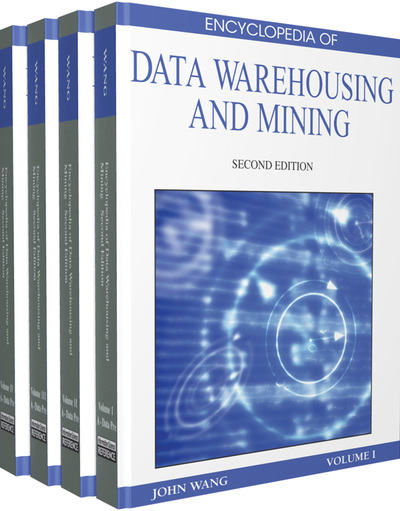# Classification of Graph Structures

Andrzej Dominik (Warsaw University of Technology, Poland)
DOI: 10.4018/978-1-60566-010-3.ch033

## Abstract

Classification is a classical and fundamental data mining (machine learning) task in which individual items (objects) are divided into groups (classes) based on their features (attributes). Classification problems have been deeply researched as they have a large variety of applications. They appear in different fields of science and industry and may be solved using different algorithms and techniques: e.g. neural networks, rough sets, fuzzy sets, decision trees, etc. These methods operate on various data representations. The most popular one is information system/decision table (e.g. Dominik, & Walczak, 2006) denoted by a table where rows represent objects, columns represent attributes and every cell holds a value of the given attribute for a particular object. Sometimes it is either very difficult and/or impractical to model a real life object (e.g. road map) or phenomenon (e.g. protein interactions) by a row in decision table (vector of features). In such a cases more complex data representations are required e.g. graphs, networks. A graph is basically a set of nodes (vertices) connected by either directed or undirected edges (links). Graphs are used to model and solve a wide variety of problems including classification. Recently a huge interest in the area of graph mining can be observed (e.g. Cook, & Holder, 2006). This field of science concentrates on investigating and discovering relevant information from data represented by graphs. In this chapter, we present basic concepts, problems and methods connected with graph structures classification. We evaluate performance of the most popular and effective classifiers on two kinds of classification problems from different fields of science: computational chemistry, chemical informatics (chemical compounds classification) and information science (web documents classification).
Chapter Preview
Top

## Background

There are numerous types of pattern that can be used to build classifiers. Three of these are frequent, common and contrast patterns.

Mining patterns in graph dataset which fulfill given conditions is a much more challenging task than mining patterns in decision tables (relational databases). The most computationally complex tasks are subgraph isomorphism (determining if one smaller graph is included in other larger graph) and isomorphism (testing whether any two graphs are isomorphic (really the same). The former is proved to be NP-complete while the complexity of the latter one is still not known. All the algorithms for solving the isomorphism problem present in the literature have an exponential time complexity in the worst case, but the existence of a polynomial solution has not yet been disproved. A universal exhaustive algorithm for both of these problems was proposed by Ullman (1976). It operates on the matrix representation of graphs and tries to find a proper permutation of nodes. The search space can be greatly reduced by using nodes invariants and iterative partitioning. Moreover multiple graph isomorphism problem (for a set of graphs determine which of them are isomorphic) can be efficiently solved with canonical labelling (Fortin, 1996). Canonical label is a unique representation (code) of a graph such that two isomorphic graphs have the same canonical label.

Another important issue is generating all non-isomorphic subgraphs of a given graph. The algorithm for generating DFS (Depth First Search) code can used to enumerate all subgraphs and reduce the number of required isomorphism checking. What is more it can be improved by introducing canonical labelling.

Contrast patterns are substructures that appear in one class of objects and do not appear in other classes whereas common patterns appear in more than one class of objects. In data mining, patterns which uniquely identify certain class of objects are called jumping emerging patterns (JEP). Patterns common for different classes are called emerging patterns (EP). Concepts of jumping emerging patterns and emerging patterns have been deeply researched as a tool for classification purposes in databases (Kotagiri, & Bailey, 2003). They are reported to provide high classification accuracy results. Frequent pattern is a pattern which appears in samples of a given dataset more frequently than specified threshold. Agarwal and Srikant proposed an efficient algorithm for mining frequent itemsets in the transaction database called Apriori.

## Complete Chapter List

Search this Book:
Reset